# AP 7th Class Maths Notes 5th Lesson Triangles

Students can go through AP Board 7th Class Maths Notes 5th Lesson Triangles to understand and remember the concept easily.

## AP Board 7th Class Maths Notes 5th Lesson Triangles

→ A triangle is a simple closed plane figure made up of three line segments. .

→ Based on the sides triangles are of three types.

1. Equilateral triangle
2. Isosceles triangle
3. Scalene triangle

→ Based on the angles, triangles are of three types.

1. Acute angled triangle
2. Obtuse angled triangle
3. Right angled triangle

→ The sum of the three interior angles in a triangle is 180°.

→ The exterior angle of a triangle is equal to the sum of it’s opposite interior angles.→ Properties of the lengths of the sides of a triangle:

• The sum of the lengths of any two sides of a triangle is greater than the length of the third side.
• The difference between the lengths of any two sides of a triangle is smaller than the length of the third side.

→ Triangle: A simple closed figure formed by three line segments is called a triangle. In the figure ABC is a triangle.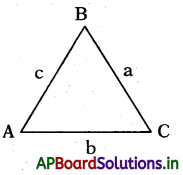• A, B, C are its corners known as vertices.
• ∠A, ∠B & ∠C are called its interior angles,
• AB, BC & CA are called its sides.
• Side opposite to ∠A is BC, also denoted by a.
• Side opposite to ∠B is AC, also denoted by b,
• Side opposite to ∠C is AB, also denoted by c.

→ Types of Triangles:
(i) Equilateral triangle: All 3 sides and angles are equal.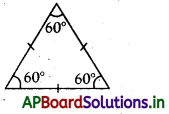(ii) Isosceles triangle: 2 equal sides and 2 equal angles.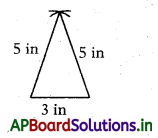(iii) Scalene triangle: Has no equal sides or angles.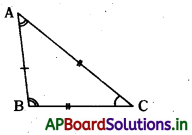(iv) Acute angled triangle: All 3 angles re acute.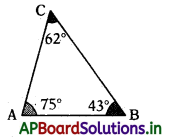(v) Right angled triangle: Has 1 right angle.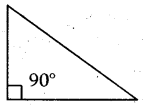(vi) Obtuse angled triangle: Has 1 Abtuse angle.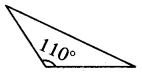Note: The sides with equal lengthifBfS! denoted by same number of strokes (/), similarly for equal angles also.

• In an equilateral triangle all the three sides and each angle is equal to 60°.
• In an isosceles triangle two sides are equal.
• In an isosceles triangle angles opposite to equal sides are equal.
• Conversely in an isosceles triangle sides opposite to equal angles are equal.
• In a right angled triangle the side opposite to right angle is called hypotenuse.
• Hypotenuse is the longest side of a right triangle.→ Angle sum property of a triangle:
The sum of three interior angles of a triangle is equal to 180°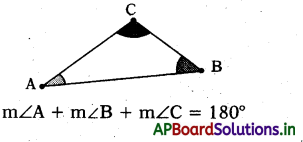∠A + ∠B + ∠C = 180°

→ Exterior angle property of a triangle:
If in a triangle any side is produced angle, then formed is called its exterior angle.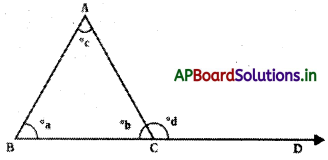In ∆ABC, BC is produced to D, then ∠ACD is called exterior angle.

→ Exterior Angle Theorem: The exterior angle of a triangle is equal to the sum of the two opposite interior angles.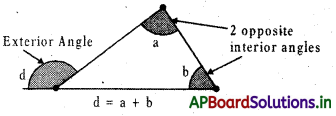Example: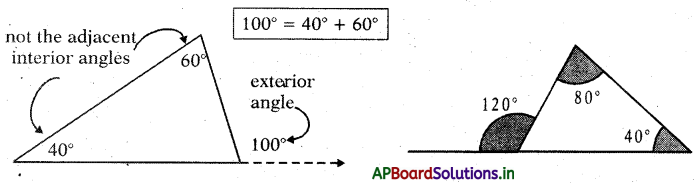→ Inequality properties of a triangle: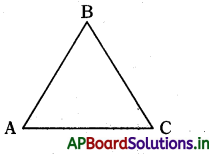→ In any ∆ABC, sum of any two sides is always greater than the third side. If AB, BC & CA are the sides of the triangle, then

• AB + BC > CA c + a > b
• BC + CA > AB a + b > c
• CA + AB > BC b + c > a

→ Also the difference between any two sides of a triangle is always less than the third side.

• AB – BC < CA c – a < b
• BC – CA < AB a – b < c
• CA – AB < BC b – c < a

→ In a triangle, if two sides are given, then the side opposite to greater angle is greater than the other.

→ In a triangle, if two sides are given, then the side opposite to smaller angle is smaller than the other.→ Also in a triangle, if two angles are given,.then the angle opposite to greater side is greater than the other.

→ In a triangle, if two angles are given, then the angle opposite to smaller side is smaller than the other.

→ The perpendicular line segment from any vertex to its opposite side is called altitude.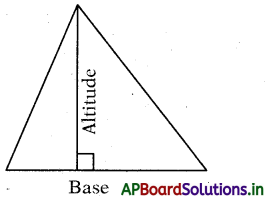Height = length of the altitude
Area = space inside the triangle